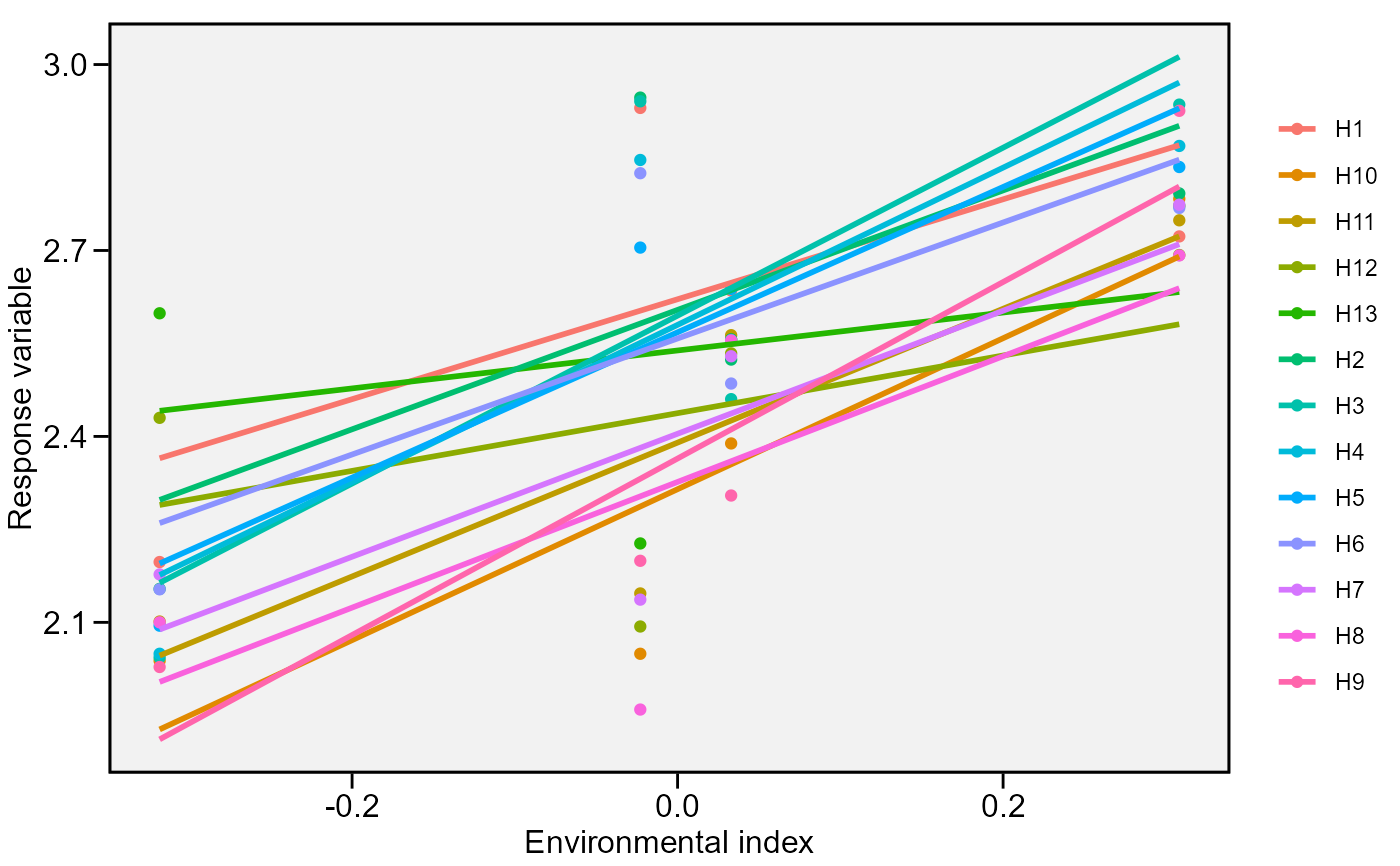Regression-based stability analysis using the Eberhart and Russell (1966) model.

ge_reg(.data, env, gen, rep, resp, verbose = TRUE)

## Arguments

.data The dataset containing the columns related to Environments, Genotypes, replication/block and response variable(s) The name of the column that contains the levels of the environments. The name of the column that contains the levels of the genotypes. The name of the column that contains the levels of the replications/blocks The response variable(s). To analyze multiple variables in a single procedure use, for example, resp = c(var1, var2, var3). Logical argument. If verbose = FALSE the code will run silently.

## Value

An object of class ge_reg with the folloing items for each variable:

• data: The data with means for genotype and environment combinations and the environment index

• anova: The analysis of variance for the regression model.

• regression: A data frame with the following columns: GEN, the genotypes; b0 and b1 the intercept and slope of the regression, respectively; t(b1=1) the calculated t-value; pval_t the p-value for the t test; s2di the deviations from the regression (stability parameter); F(s2di=0) the F-test for the deviations; pval_f the p-value for the F test; RMSE the root-mean-square error; R2 the determination coefficient of the regression.

• b0_variance: The variance of b0.

• b1_variance: The variance of b1.

Eberhart, S.A., and W.A. Russell. 1966. Stability parameters for comparing Varieties. Crop Sci. 6:36-40. doi: 10.2135/cropsci1966.0011183X000600010011x

superiority(), ecovalence(), ge_stats()

## Author

Tiago Olivoto, tiagoolivoto@gmail.com

## Examples

# \donttest{
library(metan)
reg <- ge_reg(data_ge2,
env = ENV,
gen = GEN,
rep = REP,
resp = PH)
#> Evaluating trait PH |============================================| 100% 00:00:00

plot(reg)# }